# CRYPTARITHMETIC AND LOGIC INST 4200 David J Stucki

• Slides: 17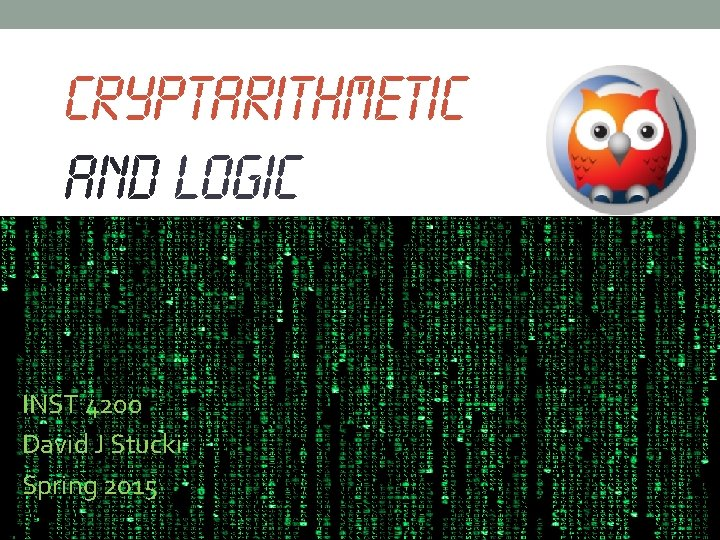CRYPTARITHMETIC AND LOGIC INST 4200 David J Stucki Spring 2015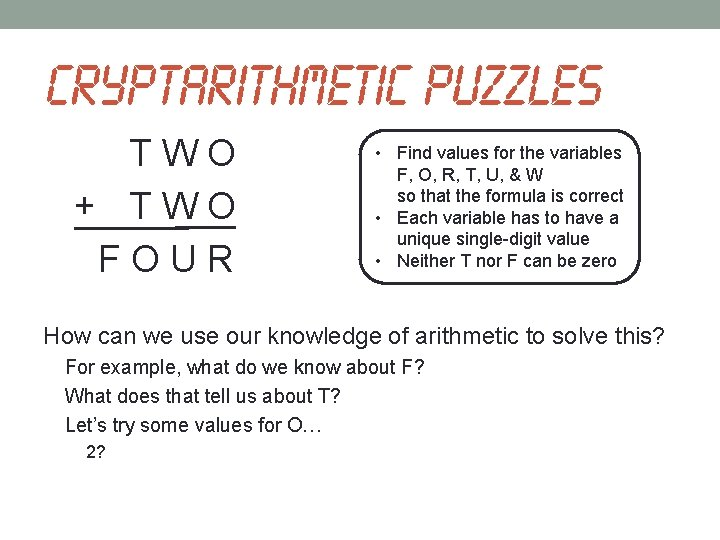Cryptarithmetic puzzles T W O + T W O F O U R • Find values for the variables F, O, R, T, U, & W so that the formula is correct • Each variable has to have a unique single-digit value • Neither T nor F can be zero How can we use our knowledge of arithmetic to solve this? For example, what do we know about F? What does that tell us about T? Let’s try some values for O… 2?Cryptarithmetic puzzles T W 2 + T W 2 F 2 U R • Find values for the variables F, O, R, T, U, & W so that the formula is correct • Each variable has to have a unique single-digit value • Neither T nor F can be zero How can we use our knowledge of arithmetic to solve this? For example, what do we know about F? What does that tell us about T? Let’s try some values for O… 2?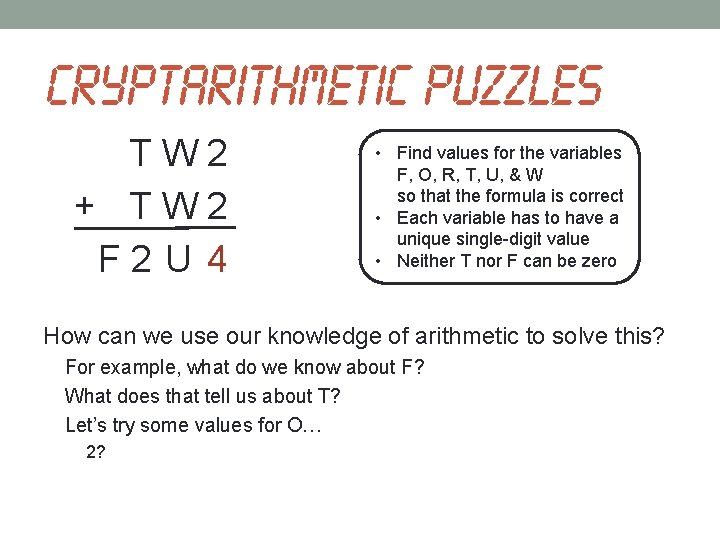Cryptarithmetic puzzles T W 2 + T W 2 F 2 U 4 • Find values for the variables F, O, R, T, U, & W so that the formula is correct • Each variable has to have a unique single-digit value • Neither T nor F can be zero How can we use our knowledge of arithmetic to solve this? For example, what do we know about F? What does that tell us about T? Let’s try some values for O… 2?Cryptarithmetic puzzles 6 W 2 + 6 W 2 1 2 U 4 • Find values for the variables F, O, R, T, U, & W so that the formula is correct • Each variable has to have a unique single-digit value • Neither T nor F can be zero How can we use our knowledge of arithmetic to solve this? For example, what do we know about F? What does that tell us about T? Let’s try some values for O… 2? NOPE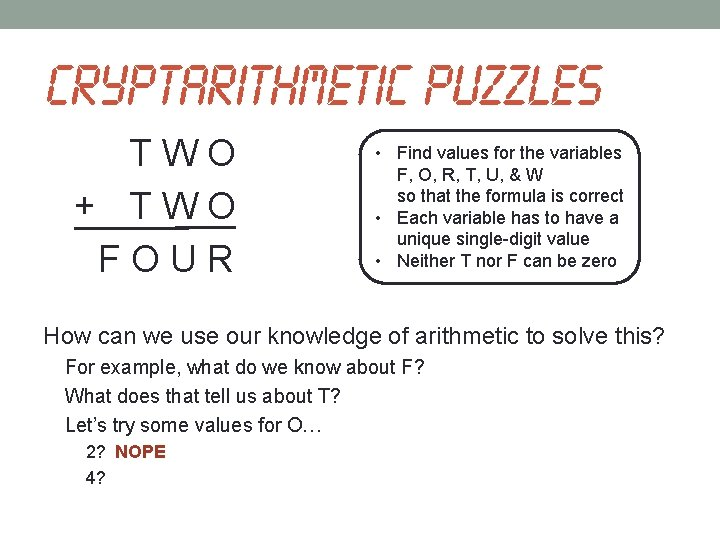Cryptarithmetic puzzles T W O + T W O F O U R • Find values for the variables F, O, R, T, U, & W so that the formula is correct • Each variable has to have a unique single-digit value • Neither T nor F can be zero How can we use our knowledge of arithmetic to solve this? For example, what do we know about F? What does that tell us about T? Let’s try some values for O… 2? NOPE 4?Cryptarithmetic puzzles T W 4 + T W 4 F 4 U R • Find values for the variables F, O, R, T, U, & W so that the formula is correct • Each variable has to have a unique single-digit value • Neither T nor F can be zero How can we use our knowledge of arithmetic to solve this? For example, what do we know about F? What does that tell us about T? Let’s try some values for O… 2? NOPE 4?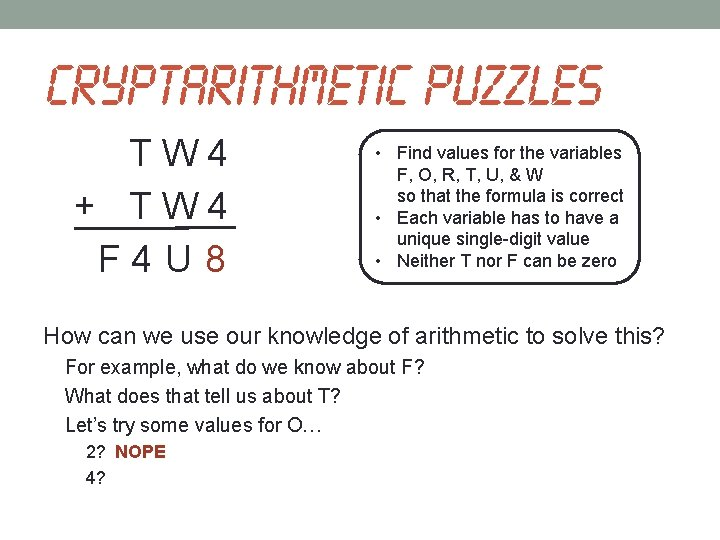Cryptarithmetic puzzles T W 4 + T W 4 F 4 U 8 • Find values for the variables F, O, R, T, U, & W so that the formula is correct • Each variable has to have a unique single-digit value • Neither T nor F can be zero How can we use our knowledge of arithmetic to solve this? For example, what do we know about F? What does that tell us about T? Let’s try some values for O… 2? NOPE 4?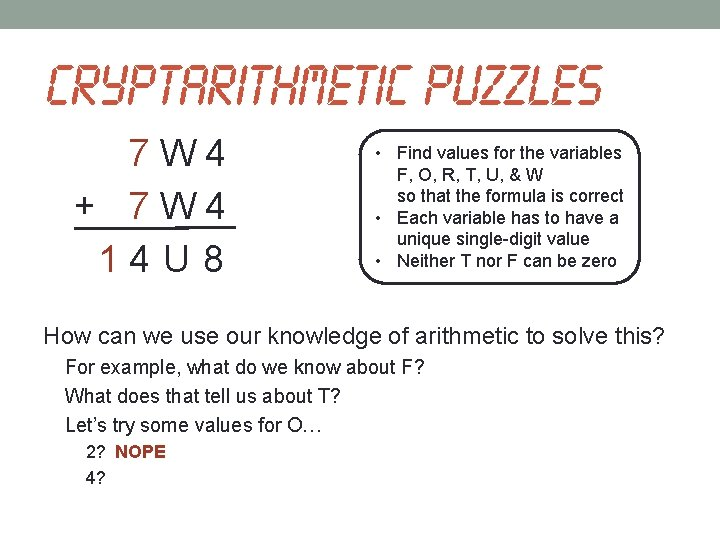Cryptarithmetic puzzles 7 W 4 + 7 W 4 1 4 U 8 • Find values for the variables F, O, R, T, U, & W so that the formula is correct • Each variable has to have a unique single-digit value • Neither T nor F can be zero How can we use our knowledge of arithmetic to solve this? For example, what do we know about F? What does that tell us about T? Let’s try some values for O… 2? NOPE 4?Cryptarithmetic puzzles 7 3 4 + 7 3 4 1 4 6 8 • Find values for the variables F, O, R, T, U, & W so that the formula is correct • Each variable has to have a unique single-digit value • Neither T nor F can be zero How can we use our knowledge of arithmetic to solve this? For example, what do we know about F? What does that tell us about T? Let’s try some values for O… 2? NOPE 4? YUP!!!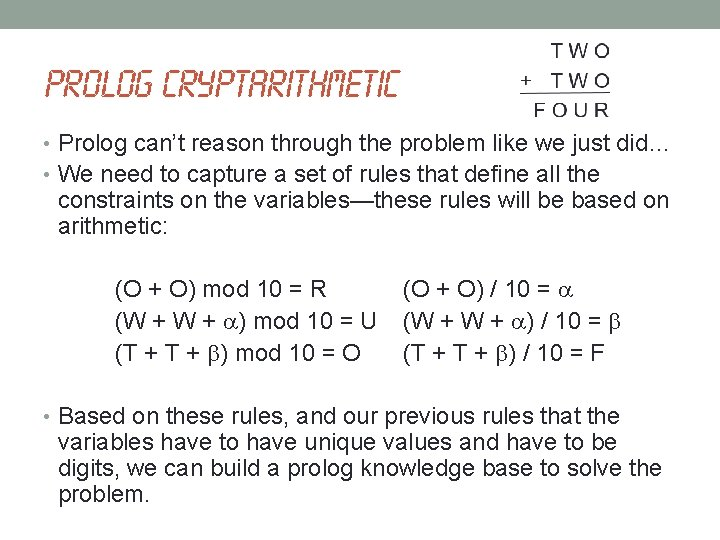Prolog Cryptarithmetic • Prolog can’t reason through the problem like we just did… • We need to capture a set of rules that define all the constraints on the variables—these rules will be based on arithmetic: (O + O) mod 10 = R (W + ) mod 10 = U (T + ) mod 10 = O (O + O) / 10 = (W + ) / 10 = (T + ) / 10 = F • Based on these rules, and our previous rules that the variables have to have unique values and have to be digits, we can build a prolog knowledge base to solve the problem.Prolog Cryptarithmetic solutions(F, O, R, T, U, W) : uniq_digits(F, O, R, T, U, W), F > 0, T > 0, R is (O + O) mod 10, Alpha is (O + O) // 10, U is (W+W+Alpha) mod 10, Beta is (W+W+Alpha) // 10, O is (T+T+Beta) mod 10, F is (T+T+Beta) // 10. uniq_digits(F, O, R, T, U, W) : dig(F), dig(O), dig(R), dig(T), dig(U), dig(W), +F=O, +F=R, +F=T, +F=U, +F=W, +O=R, +O=T, +O=U, +O=W, +R=T, +R=U, +R=W, +T=U, +T=W, +U=W. dig(0). dig(1). dig(2). dig(3). dig(4). dig(5). dig(6). dig(7). dig(8). dig(9).Prolog Cryptarithmetic • This solution is not very efficient. Why? • Because it tries to find unique values for the variables first, and then checks the other criteria. • It would be faster to only check for uniqueness when we have values that satisfy all the other constraints. • It is also more efficient to check for constraints on a variable as soon as possible after it is introduced.Prolog Cryptarithmetic solutions(F, O, R, T, U, W) : dig(O), R is (O + O) mod 10, Alpha is (O + O) // 10, dig(W), U is (W+W+Alpha) mod 10, Beta is (W+W+Alpha) // 10, dig(T), T > 0, O is (T+T+Beta) mod 10, F is (T+T+Beta) // 10, dig(F), F > 0, uniq_digits(F, O, R, T, U, W) : dig(F), dig(O), dig(R), dig(T), dig(U), dig(W), +F=O, +F=R, +F=T, +F=U, +F=W, +O=R, +O=T, +O=U, +O=W, +R=T, +R=U, +R=W, +T=U, +T=W, +U=W. dig(0). dig(1). dig(2). dig(3). dig(4). dig(5). dig(6). dig(7). dig(8). dig(9).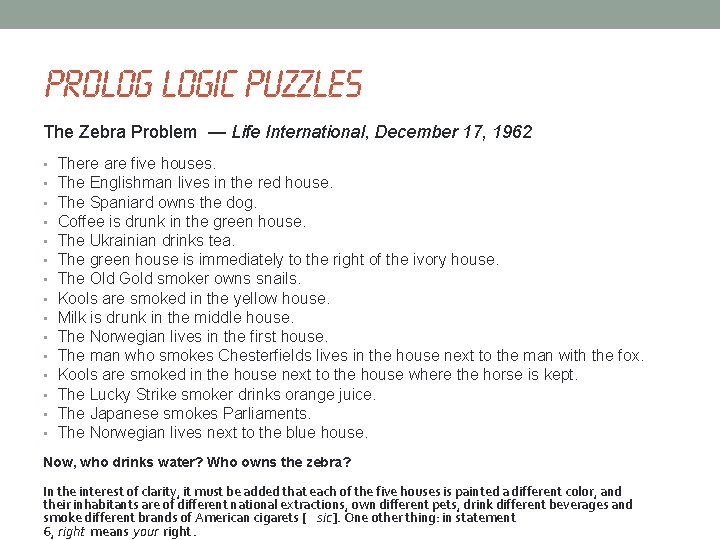Prolog Logic Puzzles The Zebra Problem — Life International, December 17, 1962 • • • • There are five houses. The Englishman lives in the red house. The Spaniard owns the dog. Coffee is drunk in the green house. The Ukrainian drinks tea. The green house is immediately to the right of the ivory house. The Old Gold smoker owns snails. Kools are smoked in the yellow house. Milk is drunk in the middle house. The Norwegian lives in the first house. The man who smokes Chesterfields lives in the house next to the man with the fox. Kools are smoked in the house next to the house where the horse is kept. The Lucky Strike smoker drinks orange juice. The Japanese smokes Parliaments. The Norwegian lives next to the blue house. Now, who drinks water? Who owns the zebra? In the interest of clarity, it must be added that each of the five houses is painted a different color, and their inhabitants are of different national extractions, own different pets, drink different beverages and smoke different brands of American cigarets [ sic]. One other thing: in statement 6, right means your right.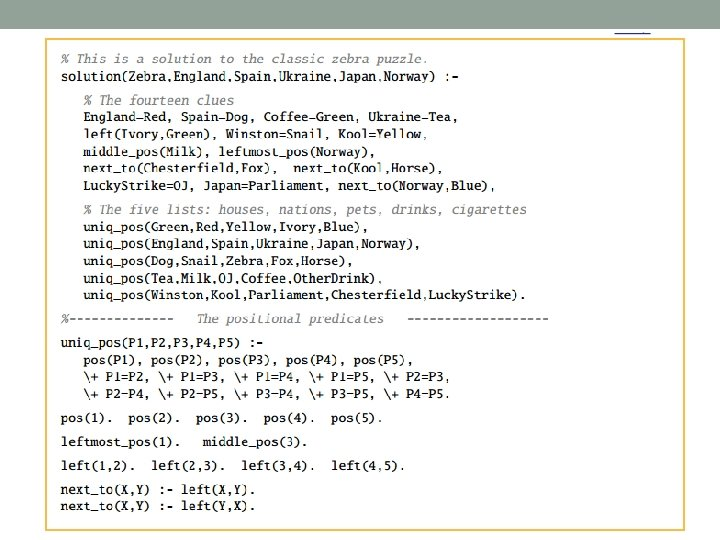NEXT TIME… • The Most Human, Chapter 7 (pp. 150 -173)